JMSLTM Numerical Library 7.2.0
com.imsl.datamining.neural

## Class Network

• ### Constructor Summary

Constructors
Constructor and Description
`Network()`
Default constructor for `Network`.
• ### Method Summary

Methods
Modifier and Type Method and Description
`double[]` ```computeStatistics(double[][] xData, double[][] yData)```
Computes error statistics.
`abstract HiddenLayer` `createHiddenLayer()`
Creates the next `HiddenLayer` in the `Network`.
`abstract double[]` `forecast(double[] x)`
Returns a forecast for each of the `Network`'s outputs computed from the trained `Network`.
`abstract double[][]` `getForecastGradient(double[] x)`
Returns the derivatives of the outputs with respect to the weights.
`abstract InputLayer` `getInputLayer()`
Returns the `InputLayer` object.
`abstract Link[]` `getLinks()`
Returns an array containing the `Link` objects in the ``` Network```.
`abstract int` `getNumberOfInputs()`
Returns the number of `Network` inputs.
`abstract int` `getNumberOfLinks()`
Returns the number of `Network` `Link`s among the `node`s.
`abstract int` `getNumberOfOutputs()`
Returns the number of `Network` output `Perceptron`s.
`abstract int` `getNumberOfWeights()`
Returns the number of weights in the `Network`.
`abstract OutputLayer` `getOutputLayer()`
Returns the `OutputLayer`.
`abstract Perceptron[]` `getPerceptrons()`
Returns an array containing the `Perceptron`s in the ``` Network```.
`abstract double[]` `getWeights()`
Returns the weights.
`abstract void` `setWeights(double[] weights)`
Sets the weights.
• ### Methods inherited from class java.lang.Object

`clone, equals, finalize, getClass, hashCode, notify, notifyAll, toString, wait, wait, wait`
• ### Constructor Detail

• #### Network

`public Network()`
Default constructor for `Network`. Since this class is abstract, it cannot be instantiated directly; this constructor is used by constructors in classes derived from `Network`.
• ### Method Detail

• #### computeStatistics

```public double[] computeStatistics(double[][] xData,
double[][] yData)```
Computes error statistics.

This is a static method that can be used to compute the statistics regardless of the training class used to train the `Network`.

Computes statistics related to the error. In this table, the observed values are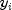. The forecasted values are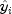. The mean observed value is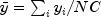, where N is the number of observations and C is the number of classes per observation.

 Index Name Formula 0 SSE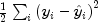1 RMS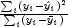2 Laplacian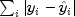3 Scaled Laplacian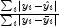4 Max residual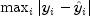Parameters:
`xData` - A `double` matrix containing the input values.
`yData` - A `double` array containing the observed values.
Returns:
A `double` array containing the above described statistics.
• #### createHiddenLayer

`public abstract HiddenLayer createHiddenLayer()`
Creates the next `HiddenLayer` in the `Network`.
Returns:
The new `HiddenLayer`.
• #### forecast

`public abstract double[] forecast(double[] x)`
Returns a forecast for each of the `Network`'s outputs computed from the trained `Network`.
Parameters:
`x` - A `double` array of values with the same length and order as the training patterns used to train the ``` Network```.
Returns:
A `double` array containing the forecasts for the output `Perceptron`s. Its length is equal to the number of output `Perceptron`s.

`public abstract double[][] getForecastGradient(double[] x)`
Returns the derivatives of the outputs with respect to the weights.
Parameters:
`x` - A `double` array which specifies the input values at which the gradient is to be evaluated.
Returns:
A `double` array containing the gradient values. The value of `gradient[i][j]` is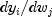, whereis the i-th output and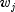is the j-th weight.
• #### getInputLayer

`public abstract InputLayer getInputLayer()`
Returns the `InputLayer` object.
Returns:
The `Network` `InputLayer`.

`public abstract Link[] getLinks()`
Returns an array containing the `Link` objects in the ``` Network```.
Returns:
An array of `Link`s associated with this ``` Network```.
• #### getNumberOfInputs

`public abstract int getNumberOfInputs()`
Returns the number of `Network` inputs.
Returns:
An `int` which contains the number of inputs.

`public abstract int getNumberOfLinks()`
Returns the number of `Network` `Link`s among the `node`s.
Returns:
An `int` which contains the number of `Link`s in the `Network`.
• #### getNumberOfOutputs

`public abstract int getNumberOfOutputs()`
Returns the number of `Network` output `Perceptron`s.
Returns:
An `int` which contains the number of outputs.
• #### getNumberOfWeights

`public abstract int getNumberOfWeights()`
Returns the number of weights in the `Network`.
Returns:
An `int` which contains the number of weights associated with this `Network`.
• #### getOutputLayer

`public abstract OutputLayer getOutputLayer()`
Returns the `OutputLayer`.
Returns:
The `Network` `OutputLayer`.
• #### getPerceptrons

`public abstract Perceptron[] getPerceptrons()`
Returns an array containing the `Perceptron`s in the ``` Network```.
Returns:
An array of `Perceptron`s associated with this ``` Network```.
• #### getWeights

`public abstract double[] getWeights()`
Returns the weights.
Returns:
A `double` array containing the weights associated with `Network` `Link`s.
• #### setWeights

`public abstract void setWeights(double[] weights)`
Sets the weights.
Parameters:
`weights` - A `double` array which specifies the weights to be associated with `Network` `Link`s.
JMSLTM Numerical Library 7.2.0